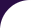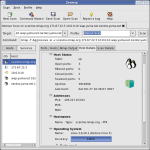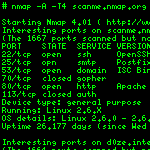Intro Reference Guide Book Install Guide Download Changelog Zenmap GUI Docs Bug Reports OS Detection Propaganda Related Projects In the Movies In the NewsLibrary tableaux

Auxiliary functions for table manipulation

Author:

• Daniel Miller

Functions

 contains (t, item, array) Check for the presence of a value in a table invert (t) Invert a one-to-one mapping keys (t) Returns the keys of a table as an array shallow_tcopy (t) Copy one level of a table. tcopy (t) Recursively copy a table.

Functions

contains (t, item, array)

Check for the presence of a value in a table

Parameters

• t: the table to search into
• item: the searched value
• array:

Return values:

1. Boolean true if the item was found, false if not
2. The index or key where the value was found, or nil
invert (t)

Invert a one-to-one mapping

Parameters

• t: the table to invert

Return value:

an inverted mapping
keys (t)

Returns the keys of a table as an array

• t: The table

Return value:

A table of keys
shallow_tcopy (t)

Copy one level of a table.

Iterates over the keys of a table and copies their values into a new table. If any values are tables, they are copied by reference only, and modifying the copy will modify the original table value as well.

Parameters

• t: the table to copy

Return value:

a shallow copy of the table
tcopy (t)

Recursively copy a table.

Uses simple assignment to copy keys and values from a table, recursing into subtables as necessary.

Parameters

• t: the table to copy

Return value:

a deep copy of the table